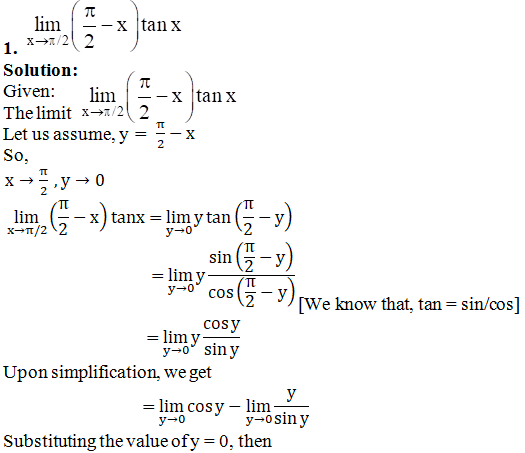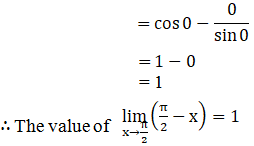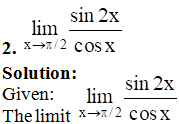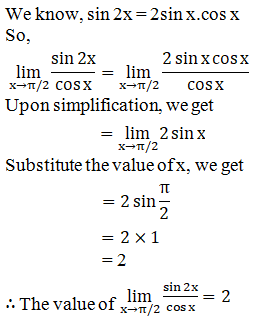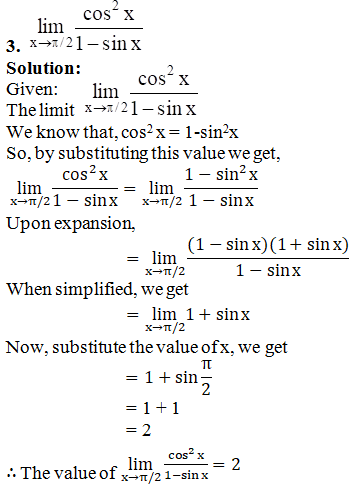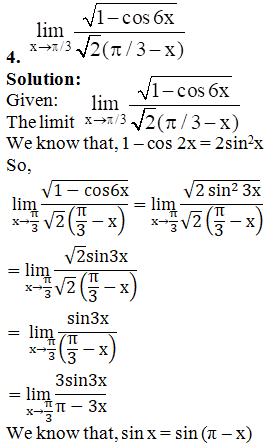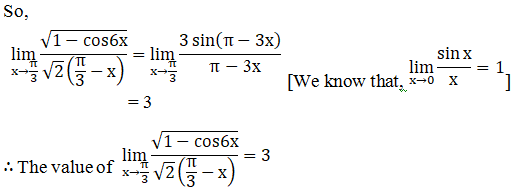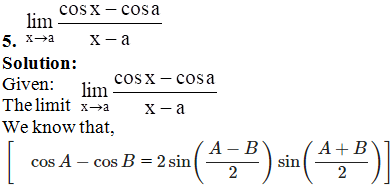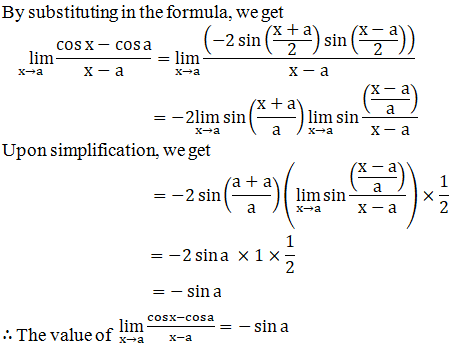# RD Sharma Solutions for Class 11 Chapter 29 - Limits Exercise 29.8

In Exercise 29.8, we shall study evaluation of trigonometric limits when the variable tends to non-zero quantity, with suitable examples to understand the concepts. Students can refer to RD Sharma Solutions, which is the best reference guide prepared by the subject experts at BYJU’S. To develop a strong command over the subject of Maths, students can use this guide to excel in exams. All the solutions are prepared by experts to match the level of all students. The pdf of RD Sharma Class 11 Maths Solutions Chapter 29 Exercise 29.8 is readily available for download, from the links given below.

## Download the pdf of RD Sharma Solutions for Class 11 Maths Exercise 29.8 Chapter 29 – Limits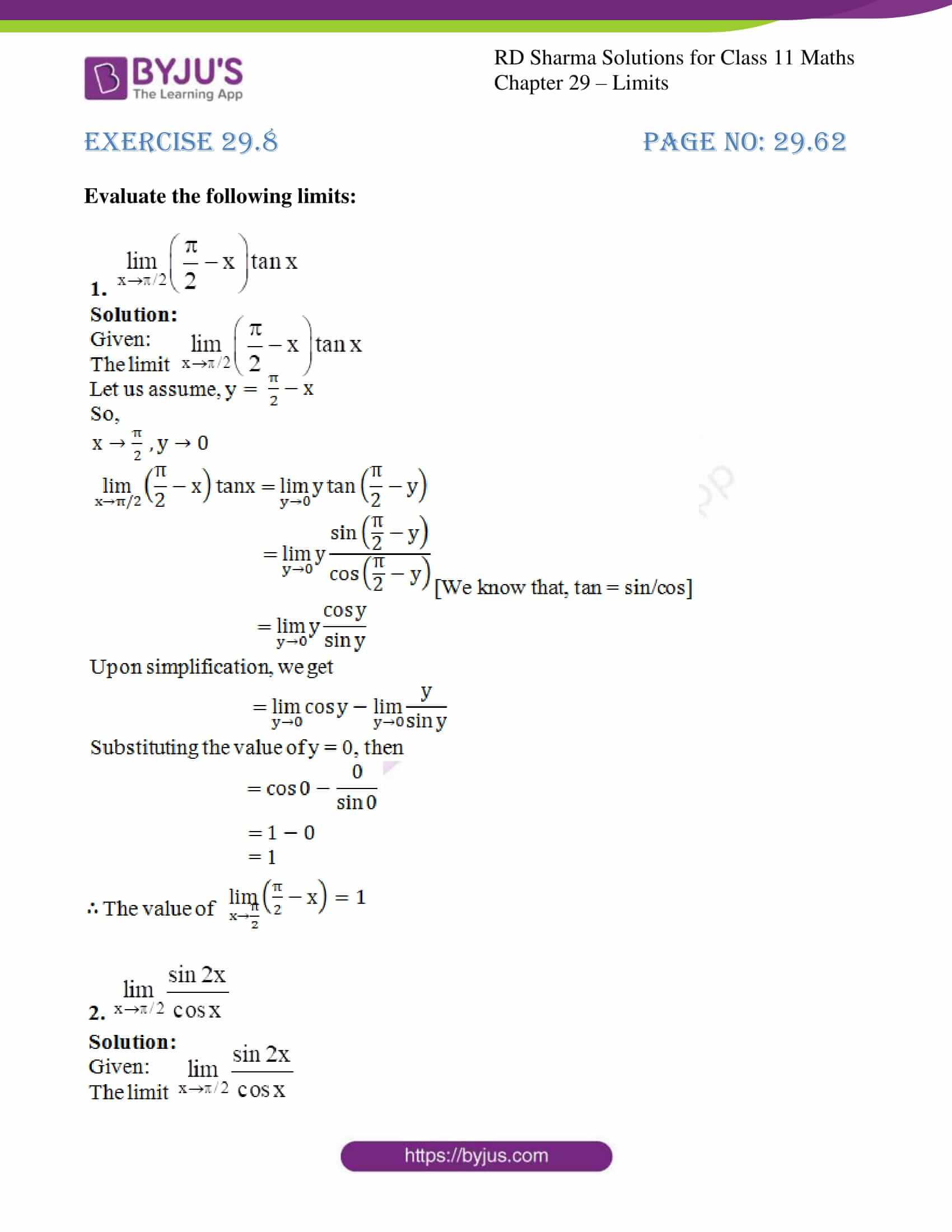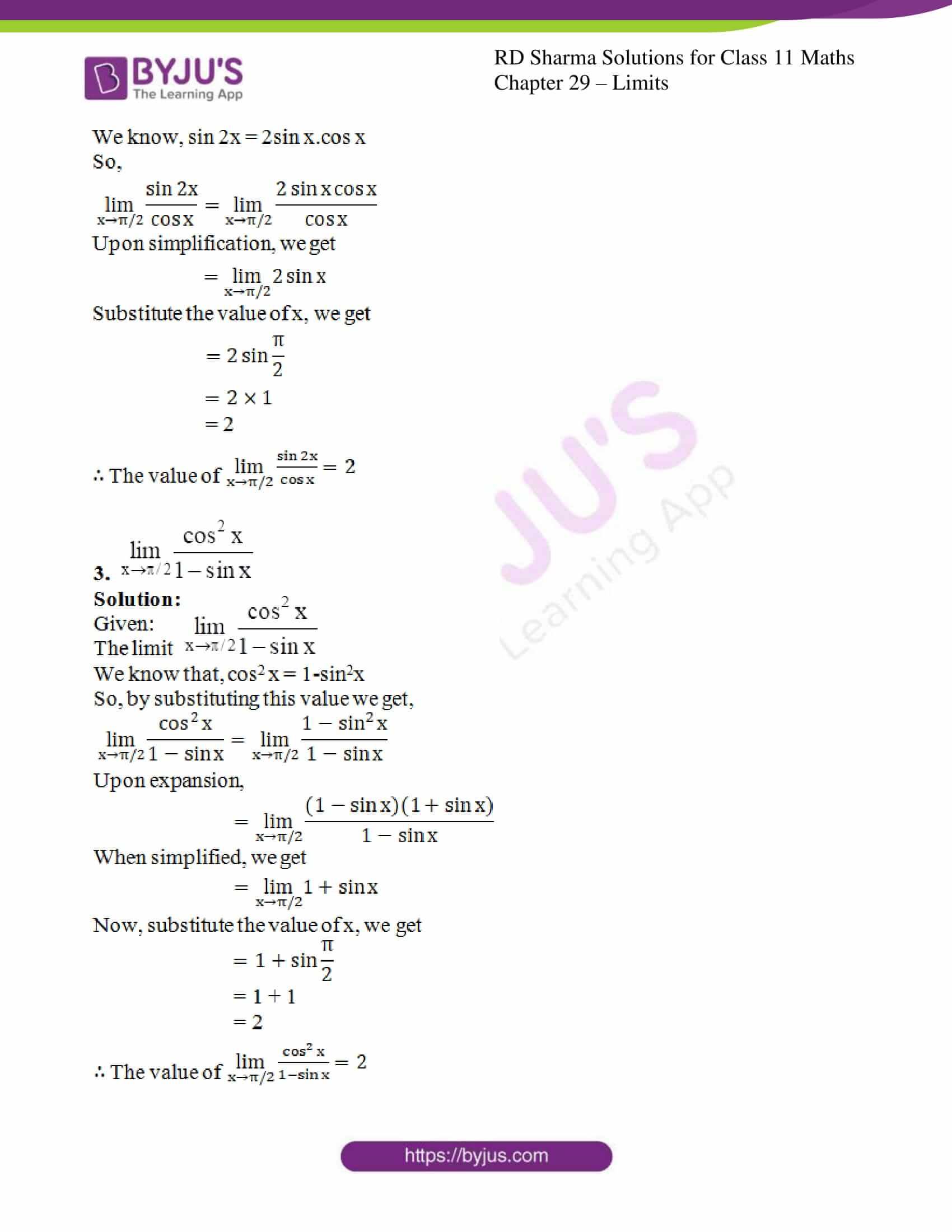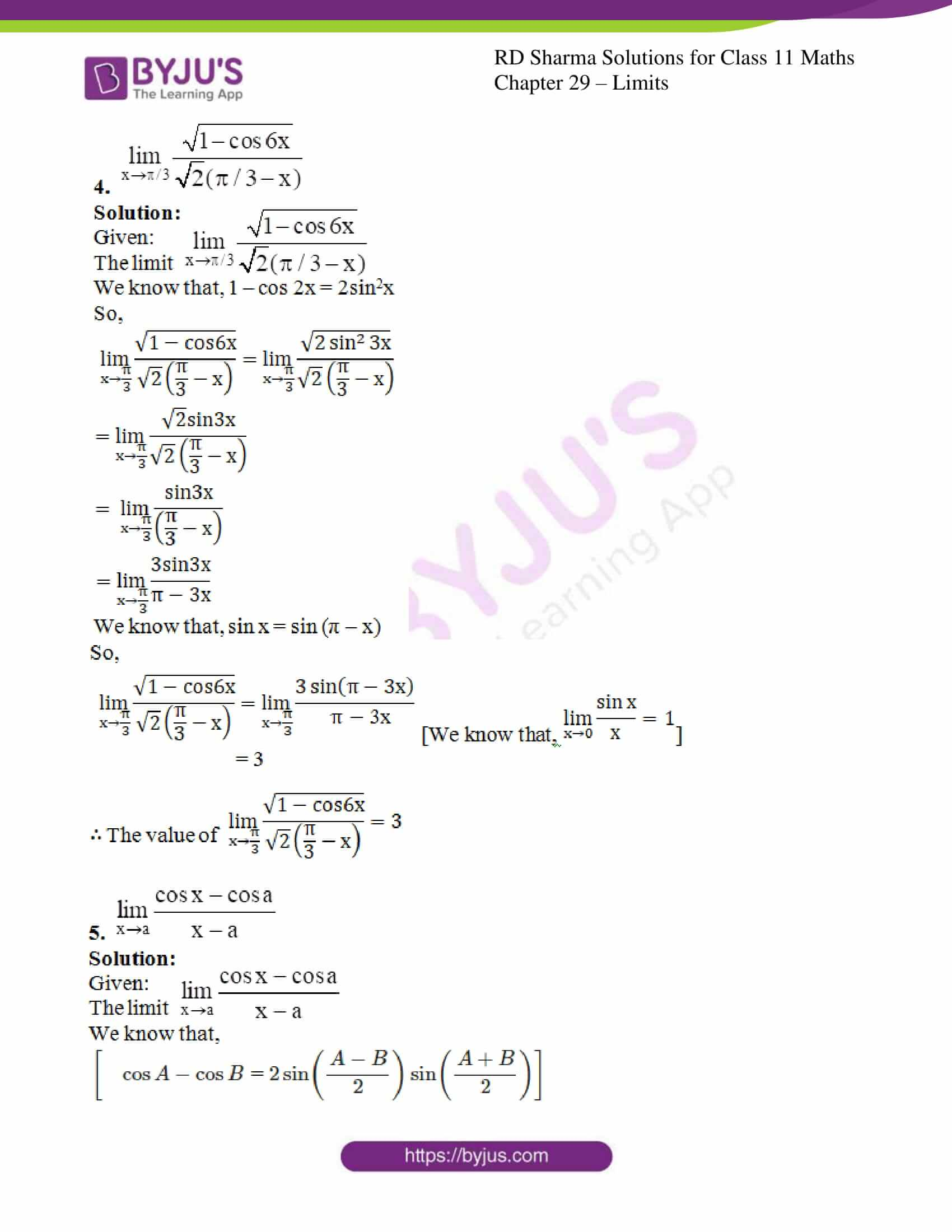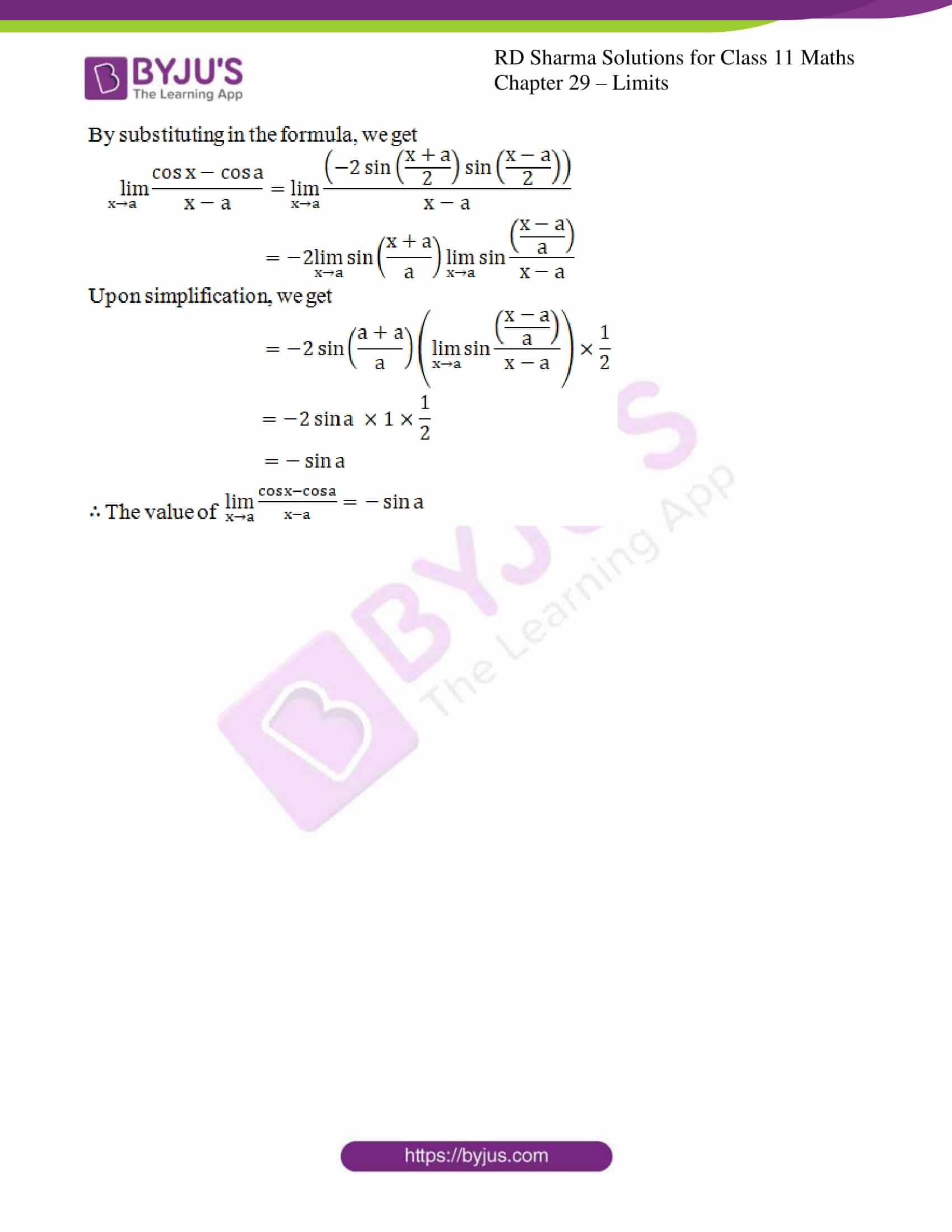### Also, access other exercises of RD Sharma Solutions for Class 11 Maths Chapter 29 – Limits

Exercise 29.1 Solutions

Exercise 29.2 Solutions

Exercise 29.3 Solutions

Exercise 29.4 Solutions

Exercise 29.5 Solutions

Exercise 29.6 Solutions

Exercise 29.7 Solutions

Exercise 29.9 Solutions

Exercise 29.10 Solutions

Exercise 29.11 Solutions

### Access answers to RD Sharma Solutions for Class 11 Maths Exercise 29.8 Chapter 29 – Limits

#### EXERCISE 29.8 PAGE NO: 29.62

Evaluate the following limits: